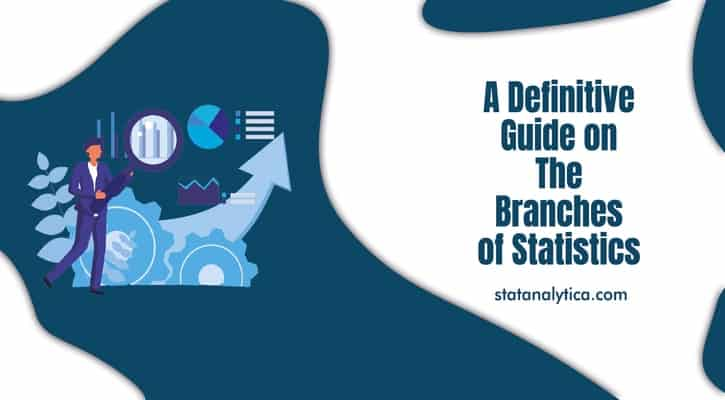# A Definitive Guide on The Branches of Statistics

Today I am going to tell you about the branches of statistics. Before we are getting started. Let’s have a quick look at what is statistics?

Statistics is the main branch of mathematics. Used to perform different operations, i.e., Data collection, organization, analysis, and so on. In other words, statistics are a form of mathematical analysis that uses quantitative models to give a set of experimental data or studies of real life. Statistics examine the methodology for collecting, reviewing, analyzing, and making data conclusions. Some statistical measures include the following:

• Mean
• Regression analysis
• Skewness
• Kurtosis
• Variance
• Analysis of variance
• Descriptive Statistics

## Branches of Statistics

### Descriptive Statistics

Descriptive statistics is the first part of statistics that deals with the collection of data. People seem it too easy, but it is not that easy. The statisticians need to be aware of the designing and experiments. They also need to choose the right focus group and avoid biases. In contrast, Descriptive statistics are used in use to do various kinds of analysis on different studies.

Descriptive statistics have two parts

• Central tendency measures
• Variability measures

To help understand the analyzed data, the tendency measures and variability measures use tables, general discussions, and charts.

#### Measures of Central Tendency

Central tendency measures specifically help statisticians evaluate the distribution center of values. These tendency measures are:

Mean

Mean is a conventional method used to describe the central tendency. Typically, to calculate the average of values, count all values, and then divide them with the number of available values.

Median

It is the result that is in the middle of a set of values. An easy way to calculate the median is to edit the results in numerical journals and locate the result that is in the center of the distributed sample.

Mode

The mode is the frequently occurring value in the given data set.

#### Measures of Variability

The variability measure helps statisticians to analyze the distribution that is spreading from a specific data set. Some of the variables of variability include quartiles, ranges, variances, and standard deviation.

### Inferential Statistics

The inference statistics are techniques that enable statisticians to use the information collected from the sample to conclude, bring decisions, or predict a defined population.

Inference statistics often speak in terms of probability by using descriptive statistics. Besides, these techniques are used primarily by a statistician for data analysis, drafting, and making conclusions from limited information. That is obtained by taking samples and testing how reliable they are.

Most predictions of the future and generalization on a population study of a smaller specimen are in the scope of the inference statistics. Besides, most of the social sciences experiments deal with the study of a small sample population that helps determine the behavior of the community.

Designing a real experiment, the researcher can bring conclusions relevant to his study. When making conclusions, it should be cautious not to draw wrongly or biased

### Different types of inferential statistics include:

• Regression analysis
• Analysis of variance (ANOVA)
• Analysis of covariance (ANCOVA)
• Statistical significance (t-test)
• Correlation analysis

## Conclusion

Now you may have a better idea about the branches of statistics. Remember that we are not digging too much into the topic. Besides this is just a beginner guide on the branches of statistics. if you are a student of statistics.

And you need statistics homework help then we are here to help you. Our experts are always there to provide you the best statistics homework help services as per your requirements and pocket-friendly charges. So what are you waiting for? Submit your work now to get instant help.

5 1 vote
Article Rating
TitleArticle
A Definitive Guide on The Branches of Statistics
Description
Statistics is the main branch of mathematics. Used to perform different operations, i.e., Data collection, organization, analysis, and so on. In other words, statistics are a form of mathematical analysis that uses quantitative models to give a set of experimental data or studies of real life.
Author
Publisher
Statanalytica
Publisher Logo
Subscribe
Notify of1 Comment
Inline Feedbacks